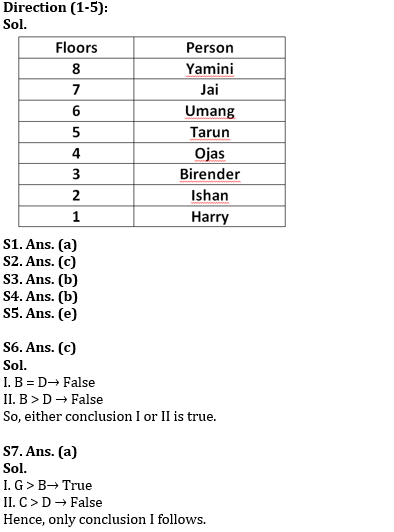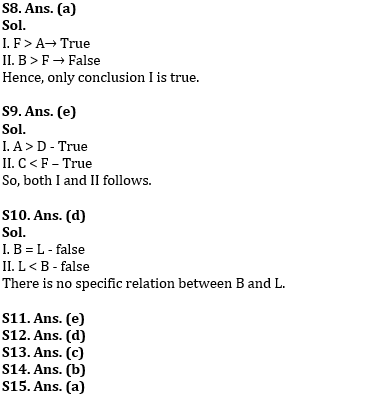Latest Banking jobs   »   Reasoning Ability Quiz For RBI Grade...

# Reasoning Ability Quiz For RBI Grade B/ ECGC PO/ SIDBI Grade A Prelims 2022- 22nd April

Directions (1-5): Study the information carefully and answer the questions given below.
Eight persons Birender, Ishan, Harry, Umang, Jai, Ojas, Tarun and Yamini are living on eight different floors of a building but not necessarily in the same order. The lowermost floor is numbered as 1, the one above floor is numbered as 2 and so on till the topmost floor is numbered as 8.
If the place value of the first letter of person’s name is even as per English alphabetical order, then they live on odd numbered floor or else live on even numbered floor. Two persons live between Jai and Ojas who does not live above Tarun. Umang lives above Birender. Two persons live between Yamini and Tarun. Tarun lives below Yamini but not on floor number 1. Ojas lives above Harry. Number of persons live below Birender are same as the number of Persons lives above Umang. Harry does not live immediately above or immediately below Yamini. Ishan live below Ojas.

Q1. On which of the following floors does Harry live?
(a) 1
(b) 2
(c) 3
(d) 4
(e) 5

Q2. How many floors are below Tarun’s floor?
(a) Six
(b) Two
(c) Four
(d) Five
(e) None of these

Q3. Ishan lives how many floors below Jai’s floor?
(a) Three
(b) Five
(c) Four
(d) Six
(e) None of these

Q4. How many floors are there between Yamini and Ojas?
(a) Two
(b) Three
(c) Four
(d) One
(e) None of these

Q5. Which person lives immediately above Umang?
(a) Harry
(b) Tarun
(c) Birender
(d) Ojas
(e) None of these

Directions (6-10): In the following questions assuming the given statement to be true, find which of the conclusion(s) among given conclusions is/are definitely true and then give your answers accordingly.

Q6. Statements: A > B ≥ C ≥ D, E = F > G, G = A
Conclusions:
I. B = D
II. B > D

(a) Only I is true
(b) Only II is true
(c) Either I or II is true
(d) Neither I nor II is true
(e) Both I and II are true

Q7. Statements: A > B ≥ C ≥ D, E = F > G, G = A
Conclusions:
I. G > B
II.C > D

(a) Only I is true
(b) Only II is true
(c) Either I or II is true
(d) Neither I nor II is true
(e) Both I and II are true

Q8. Statements: A > B ≥ C ≥ D, E = F > G, G = A
Conclusions:
I. F > A
II.B > F

(a) Only I is true
(b) Only II is true
(c) Either I or II is true
(d) Neither I nor II is true
(e) Both I and II are true

Q9. Statements: A > B > C = D < E < F
Conclusions:
I. A > D
II. C < F

(a) Only I is true
(b) Only II is true
(c) Either I or II is true
(d) Neither I nor II is true
(e) Both I and II are true

Q10.Statements: A > B, P > L, L = S
Conclusions:
I. B = L
II. L < B

(a) Only I is true
(b) Only II is true
(c) Either I or II is true
(d) Neither I nor II is true
(e) Both I and II are true

Directions (11-15): Study the following arrangement and answer the given questions:

A @ X V 4 D * L # B H 9 & X 2 B K % R 3 5 R J O 7 M ^ A 6

Q11. If all the symbols are dropped from the above arrangement, which of the following will be sixth from the right end?
(a) M
(b) A
(c) 6
(d) R
(e) J

Q12. How many such vowels are there in above arrangement, each of which is immediately preceded by a consonant and immediately followed by a number?
(a) None
(b) Three
(c) Two
(d) One
(e) None of These

Q13. How many such numbers are there in above arrangement, each of which is immediately preceded and also immediately followed by consonant?
(a) One
(b) Three
(c) Two
(d) None
(e) None of These

Q14. Which of the following will be fourth to the left of seventeenth from left end of the above arrangement?
(a) 9
(b) &
(c) X
(d) 2
(e) None of These

Q15. Which of the following is fourth to the right of eleventh to the right of first X (from left) in the given arrangement?
(a) %
(b) K
(c) B
(d) R
(e) None of These

Solutions#### Congratulations!Download Hindu Review of October 2021: Free PDF